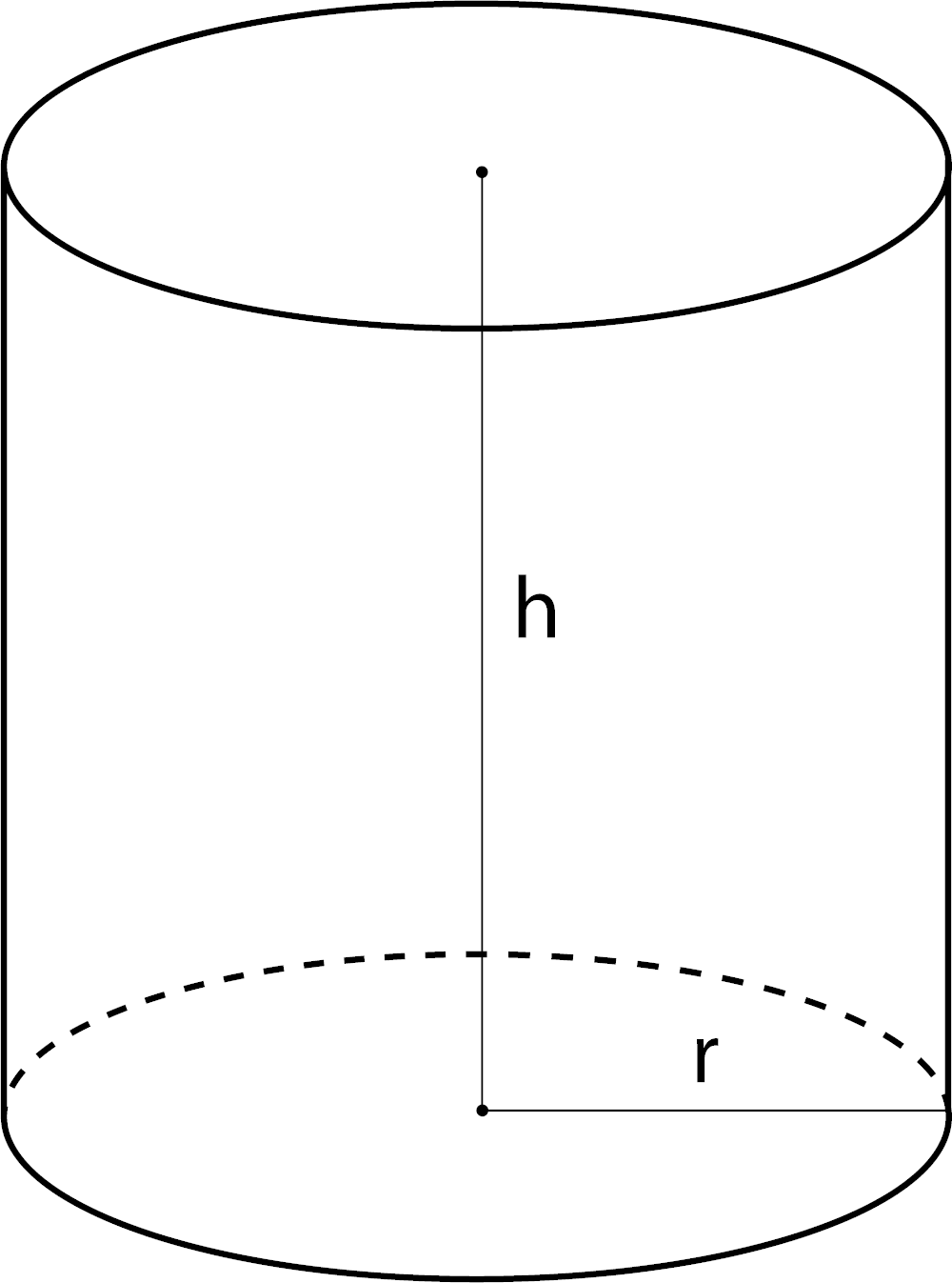# Cylinder Cubic Footage Calculator

Enter the diameter and height to calculate the cubic footage of a cylinder.

## Result:

Cubic Feet
Learn how we calculated this below

## How to Find the Volume of a Cylinder in Cubic Feet

Cubic footage is the measure of the volume of a space in cubic feet. To find the cubic footage of a cylinder, use the following formula:

volume = π × r2 × h

The volume of a cylinder in cubic feet is equal to π times the square of the base’s radius r in feet squared times the cylinder’s height h in feet.

π is roughly equal to 3.14159265359.

π = 3.14159265359

If you know the diameter of the cylinder, you can find the radius by dividing the diameter by two, or just use our circle diameter calculator.